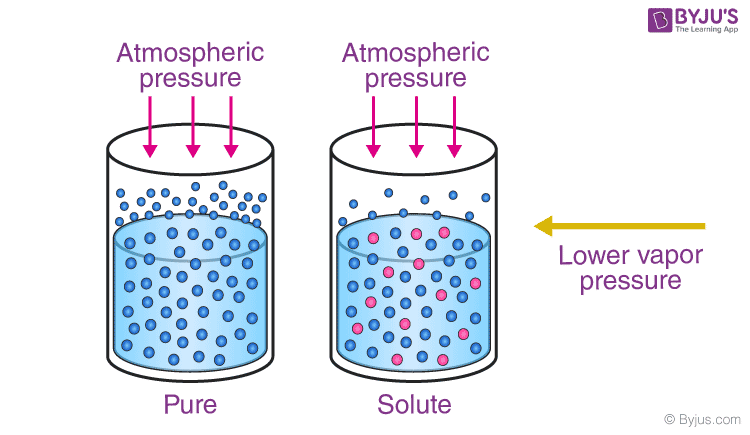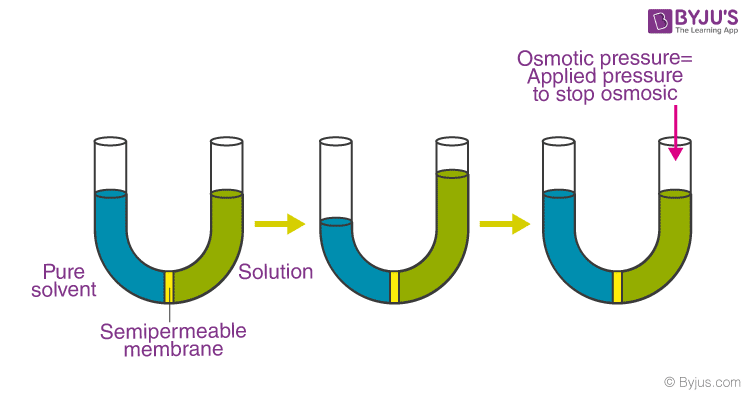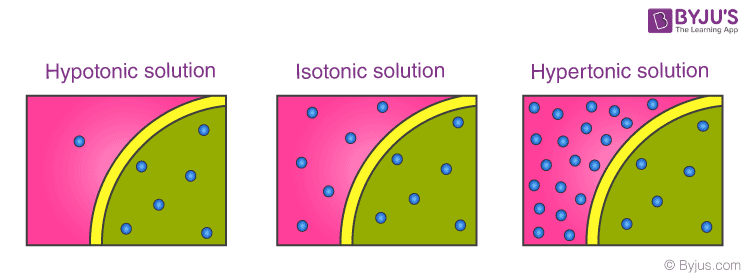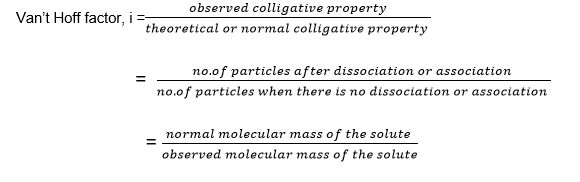Win up to 100% scholarship on Aakash BYJU'S JEE/NEET courses with ABNAT Win up to 100% scholarship on Aakash BYJU'S JEE/NEET courses with ABNAT

# Colligative Properties

A colligative property is a property of a solution that is dependent on the ratio between the total number of solute particles (in the solution) to the total number of solvent particles. Colligative properties are not dependent on the chemical nature of the solution’s components. Thus, colligative properties can be linked to several quantities that express the concentration of a solution, such as molarity, normality, and molality. The four colligative properties that can be exhibited by a solution are given below:

• Boiling point elevation
• Freezing point depression
• Relative lowering of vapour pressure
• Osmotic pressure

The word “colligative” has been adapted or taken from the Latin word “colligatus” which means “bound together”. In the context of defining a solution, colligative properties help us understand how the properties of the solution are linked to the concentration of solute in the solution.

## What Are Colligative Properties?

A dilute solution containing non-volatile solute exhibits some properties which depend only on the number of solute particles present and not on the type of solute present. These properties are called colligative properties, and they are mostly seen in dilute solutions.

We can further consider colligative properties as those properties that are obtained by the dissolution of a non-volatile solute in a volatile solvent. Generally, the solvent properties are changed by the solute, where its particles remove some of the solvent molecules in the liquid phase. This also results in the reduction of the concentration of the solvent.

Besides, when we talk about the given solute-solvent mass ratio, colligative properties are said to be inversely proportional to the solute molar mass.

## Colligative Properties Examples

We can observe the colligative properties of solutions by going through the following examples. If we add a pinch of salt to a glass full of water, its freezing temperature lowers considerably than the normal temperature. Alternatively, its boiling temperature will also increase, and the solution will have a lower vapour pressure. There will be changes in its osmotic pressure as well.

Similarly, if we add alcohol to water, the solution’s freezing point goes down below the normal temperature, and that is observed for either pure water or alcohol.

## Different Types of Colligative Properties of Solution

There are different types of colligative properties of a solution, which include vapour pressure lowering, boiling point elevation, freezing point depression and osmotic pressure.

### 1. Lowering of Vapour Pressure

In a pure solvent, the entire surface is occupied by the molecules of the solvent. If a non-volatile solute is added to the solvent, the surface now has both solute and solvent molecules; thereby fraction of the surface covered by solvent molecules gets reduced. Since the vapour pressure of the solution is solely due to the solvent alone, at the same temperature, the vapour pressure of the solution is found to be lower than that of the pure solvent.If P0 is the vapour pressure of pure solvent and Ps is the vapour pressure of the solution, the difference Po – Ps is termed as the lowering in vapour pressure. The ratio, Po – Ps / Po, is known as the relative lowering of vapour pressure.

Raoult, in 1886, established a relation between relative lowering in vapour pressure and mole fraction. The relationship is known as Raoult’s law. It states that the relative lowering in vapour pressure of a dilute solution is equal to the mole fraction of the solute present in the solution.

If n moles of solute is dissolved in N moles of the solvent, then, according to Raoult’s law,

Po – Ps / Po = n / n + N

### 2. Elevation in Boiling Point

The boiling point of a liquid is the temperature at which the vapour pressure is equal to atmospheric pressure. We know that with the addition of a non-volatile liquid to a pure solvent, the vapour pressure of a solution decrease. Therefore, to make vapour pressure equal to atmospheric pressure, we have to increase the temperature of the solution. The difference in the boiling point of the solution and the boiling point of the pure solvent is termed elevation in boiling point.

If T0b is the boiling point of the pure solvent and Tb is the boiling point of the solution, then elevation in boiling point is given as

∆Tb =T0b-Tb

Experimental results show that there is a relation between elevation in boiling point and molality ‘m’ of the solute present in the solution.

∆Tb ∝ m

∆Tb = kb m

Where,

kb = molal elevation constant

Substituting the value of ‘m’ in the above relation, we get

∆Tb = 1000 x kb x m2 / M2 x m1

Where,

m2 = mass of solvent in g

M1 = mass of solvent in kg

M2 = molar mass of solute

### 3. Depression in Freezing Point

The freezing point of a substance may be defined as the temperature at which the vapour pressure of the substance in its liquid phase is equal to its vapour pressure in the solid phase. According to Raoult’s law, when a non-volatile solid is added to the solvent, its vapour pressure decreases, and now it would become equal to that of a solid solvent at a lower temperature. The difference between the freezing point of the pure solvent and its solution is called depression in freezing point.

If T0f is the freezing point of the pure solvent and Tf is the freezing point when a non-volatile solute is dissolved in it, then depression in the freezing point is given as

∆Tf =T0f -Tf

Just like elevation in boiling point, depression in freezing point is also directly related to molality ‘m’.

∆Tf = 1000 x kf x m2 / M2 x m1

Where,

k f = molal depression constant

m2 = mass of solvent in g

M1 = mass of solvent in kg

M2 = molar mass of solute

### 4. Osmotic Pressure

When a semipermeable membrane is placed between a solution and solvent, it is observed that solvent molecules enter the solution through the semipermeable membrane, and the volume of the solution increases. The semi-permeable membrane allows only solvent molecules to pass through it, but prevents the passage of bigger molecules like solute. This phenomenon of the spontaneous flow of solvent molecules through a semipermeable membrane from a pure solvent to a solution or from a dilute to a concentrated solution is called osmosis.

The flow of solvent molecules through the semipermeable membrane can be stopped if some extra pressure is applied from the solution side. This pressure that prevents the flow of solvent is called the osmotic pressure of the solution.Osmotic pressure is a colligative property as it depends on the number of solutes present and not on the nature of the solute. Experimentally, it was proved that osmotic pressure (⫪) is directly proportional to molarity (C) and temperature (T).

Mathematically, ℼ = CRT, where R is the gas constant.

= (n2/V) RT

Here, V is the volume of solution in litres, and n2 is moles of solute

If m2 is the weight of solute and M2 molar mass of solute, then n2= m2/M2

= W2 RT / M2V

Thus, by knowing the values of ,w2, T and V, we can calculate the molar mass of the solute.

Different Solutions

• Isotonic solution: Two solutions having the same osmotic pressure at a given temperature are known as isotonic solutions. When such solutions are separated by a semi-permeable membrane, then there is no osmosis.
• Hypotonic solution: A hypotonic solution has a lower osmotic pressure than that of the surrounding, i.e., the concentration of solute particles is less than that of the surrounding. If the hypotonic solution is separated by a semipermeable membrane, then water moves out of the hypotonic solution.
• Hypertonic solution: A hypertonic solution has a higher osmotic pressure than that of the surrounding, i.e., the concentration of solute particles is more than that of the surrounding. If the hypertonic solution is separated by a semipermeable membrane, then water moves inside the hypertonic solution.To determine the molar mass, the osmotic pressure method has an advantage over other methods as pressure measurement is around room temperature. It is particularly useful for the determination of the molar mass of biomolecules as they are unstable at higher temperatures.

## Van’t Hoff Factor

When the solute undergoes dissociation or association in solution, the number of particles in solution increases or decreases and thus, colligative properties change accordingly. The extent of dissociation or association of the solute in a solution can be expressed by a factor called Van’t Hoff.## Solved Problems

1. The molal elevation constant for water is 0.513o C kg mol. When 0.2 mole of sugar is dissolved in 250 g of water, calculate the temperature at which the solution boils under atmospheric pressure.

Solution:

The elevation in boiling point can be written as

∆Tb = moles of sugar x 1000 / weight of water in gram

∆Tb = 0.2 x 1000 / 250

∆Tb = 0.8

⇒ T0b-Tb = 0.8

For pure water, T0b =100oC

⇒ Tb= 0.8 + 100

=100.80 0C

2. A solution of CaCl2 was prepared by dissolving 0.0169 g in 1 kg of distilled water (molar mass of Ca2+= 41g mol and Cl= 35.5gmol). The molal depression constant of water is 2kg mol. The depression in the freezing point of the solution is:

Solution:

Van’t Hoff factor(i) of CaCl2 is 3

Kf = 2 kg mol

Given,

Mass of CaCl2 (m2) = 0.0169 g

Molar mass of CaCl2( M2) =112 g

Weight of water(m1) =1000 g

∆Tf = i x 1000X Kf x m2 / M2 x m1

= 3 x 1000 x 2 x 0.0169 / 112 x 1000 g

= 9× 10-4

3. Hexane and heptane were mixed to form an ideal solution. At 1000C, the vapour pressure of two liquids (Hexane and heptane) are 190 kPa and 45 kPa, respectively. What will be the vapour pressure of the solution obtained by mixing 25 g of hexane and 35 g of heptane?

Solution :

No of moles of hexane, n1= 25/86= 0.29

No of moles of heptane, n2=35/100=0.35

𝜒1 = n1 / n1 + n2

𝜒1 = 0.29 / 0.29 +0.35

𝜒1 = 0.45

𝜒2 = 1 – 0.45

𝜒2 = 0.55

P = P01𝜒1 +P02𝜒2

= 1900.45 + 450.55

= 110 kPa

4. Which of the following effects is observed on adding non-volatile solute to a solvent?

a. Increase in vapour pressure

b. Increase in freezing point

c. Decrease in osmotic pressure

d. Decrease in vapour pressure

Solution: Option d

In a pure solvent, the entire surface is occupied by the molecules of the solvent. If a non-volatile solute is added to the solvent, the surface now has both solute and solvent molecules; thereby fraction of the surface covered by solvent molecules gets reduced. Since the vapour pressure of the solution is solely due to the solvent alone, at the same temperature, the vapour pressure of the solution is found to be lower than that of the pure solvent.

5. 300 cm3 of an aqueous solution contains 1.56 g of a polymer. The osmotic pressure of such a solution at 270oC is found to be 2.57 ✕ 10-3 bar. Calculate the molar mass of the polymer.

Solution:

Weight of polymer ( W2) = 1.56g

Osmotic pressure (⫪) = 2.57 ✕ 10-3 bar

volume (V) = 300 cm3 = 0.3L

M2 = W2 RT / V

= 1.56 x 0.083 x 300 / 0.3 x 2.57 x 10-3

= 50381 g mol

## Colligative Properties – Part 1## Colligative Properties – Part 2Q1

### Are colligative properties physical or chemical?

The physical modifications that occur from applying solute to a solution are colligative properties. Colligative properties rely on the quantities of solvent particles and the quantity of solvent, and they depend on the type of solvent, but they do NOT depend on the form of solvent particles.

Q2

### Why are colligative properties important?

Colligative properties include the lowering of vapour pressure, elevation of the boiling point, depression of the freezing point, and osmotic pressure. As mentioned in this module, this small set of properties is of fundamental significance to many natural phenomena and technical applications.

Q3

### Why is molality used in colligative properties?

Molality is used in freezing depression points for colligative properties since the colligative properties only depend on the number of particles in the solution. Molality, much like molarity, does not depend on the temperature. The volume changes slightly if the temperature changes, and the molarity will also change.

Q4

### What are the other colligative properties of a solution?

Solutions’ colligative properties are properties that depend on the concentration of molecules or ions of the solute but not on the identity of the solute. Colligative properties include lowering of vapour pressure, boiling point elevation, depression of the freezing point, and osmotic pressure.

Q5

### What does freezing point depression mean?

Depression from freezing point. The freezing point of a solution is smaller than the freezing point of a pure solvent. This suggests that for freezing to occur, a solution must be cooled to a lower temperature than the pure solvent.

Test your Knowledge on Colligative Properties